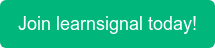What are Hazard Rates?

The hazard rate is the probability that if the event in question has not already occurred, it will occur in the next time interval, divided by the length of that interval.

The death rate for an object of a certain age is the hazard rate (x). It's part of a bigger equation called the hazard function, which looks at the chances of anything surviving to a specific point in time based on its survival at a previous point in time (t).

Example of Hazard Rates:

As a formula, the hazard ratio, defined as the relative risk of an event happening at time t, is: λ(t) / λ0

A hazard ratio of 3 means that three times the number of events are seen in the treatment group at any point in time.

Because they may be used to calculate unconditional default probabilities, hazard rates are appealing. Assume that h represents the average hazard rate from time zero to time t. Between time zero and time t, the unconditional default probability is

1 – exp(-ht)

The probability of survival (or survival rate) to time t is

exp(-ht)

Topics: ACCA, CIMA, CPD, AAT, FRM•### Central Counterparties

•### Country Risk

•### Convexity Formula

•### Clean and Dirty Price

•### Basic Indicator Approach

•### ACCA Exam Fees 2022: Everything You Need to Know

•### ACCA Exam Dates for 2022

•### How Difficult is Passing ACCA? An Honest Review

•### 7 of the Best YouTube Channels for Accounting Students

•